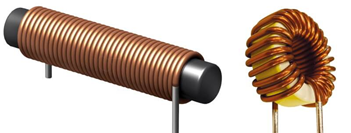## Inductance

Define the term "Inductance".Hint
The unit for electrical inductance is a henry (H), which has base units of:
$$\frac{kg\cdot m^{2}}{s^{2}\cdot A^{2}}$$\$
Electrical inductance is the tendency of an electrical conductor to oppose a change in the current flowing through it. More specifically, it is the ratio of the induced voltage to the rate of current change causing it.

Inductance is an inductor's ability to store energy in a magnetic field created by an electrical current flow. The energy required to set up the magnetic field is released when the field disappears. Due to the magnetic field associated with the flow of electrical current, inductors generate an opposing voltage that is proportional to the current change rate in a circuit, which is Inductance.
Electrical inductance is the tendency of an electrical conductor to oppose a change in the current flowing through it.